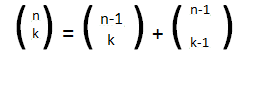Open in App
Not now

# GATE | GATE CS 1997 | Question 59

• Difficulty Level : Basic
• Last Updated : 25 Oct, 2017

Consider the following function

```Function F (n, m: integer): integer;
begin
If (n<=0) or (m<=0) then F:=1
else
F:= F(n-1,m) + F(n, m-1);
end;```

Use the recurrence relation to answer the following question.Assume that n, m are positive integers. Write only the answers without any explanation.
a. What is the value of F(n,2)?
b. What is the value of (n,m)?
c. How many recursive calls are made to the function F, including the original call, when evaluating F(n,m).